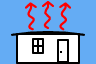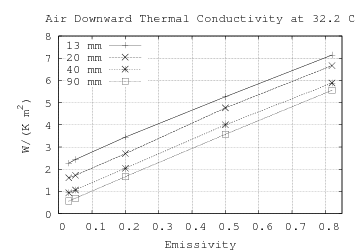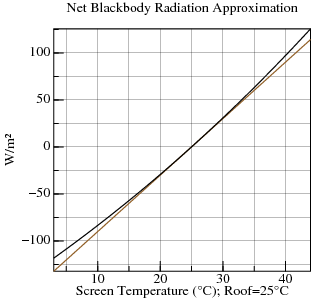http://people.csail.mit.edu/jaffer/SimRoof/SolarScreen

# SimRoof: Solar Screen

A solar screen is a film of material between the roof and sky enclosing an airspace. The screen temperature can be higher or lower than the roof temperature, depending on conditions.

A heated screen adds an additional downward thermal infrared source to the simulation. The distance between the screen and the roof (emitter) is small enough that the enclosed air doesn't interact with radiation. That means that interactions between the roof and solar screen do not need high spectral resolution, and can be computed after the NIRT model is generated.

## Definitions

symbol units description
τS screen-infrared-transmission
εB roof-emissivity
εST screen-top-emissivity
εSB screen-bottom-emissivity
AB roof-solar-absorbance
AST screen-top-solar-absorbance
ASB screen-bottom-solar-absorbance
tS screen-solar-transmission
G bounce-gain (defined below)
TA K TMY3 Dry Bulb (air) Temperature
TB K roof-temperature.K
TS K screen-temperature.K
I W/m2 TMY3 Global Horizontal Irradiance
IB W/m2 Solar Irradiance absorbed by Roof
IS W/m2 Solar Irradiance absorbed by Screen
N(TA) W/m2 Net sky-to-roof infrared radiation
k W/(m2·K) airspace-conductance
h(v) W/(m2·K) Non-emissive surface conductance (at TMY3 Wind Speed v) (see Convection)
σ W/(m2·K4) Stefan–Boltzmann constant

## Thermal Conductance of Plane Air Space

Because the roof is at or below ambient temperature, there is insignificant upward convection to the screen. That leaves radiation (treated separately) and (downward) conduction. From 1997 ASHRAE Fundamentals Handbook (SI) chapter 24 Table 3 "Thermal Resistances of Plane Air Spaces" we can extrapolate the non-emissive downward conductivity for 13 mm, 20 mm, 40 mm, and 90 mm airspaces (left graph). The graph on the right shows the curve connecting the four non-emissive resistances (reciprocal of conductance). The d/0.0266 line is the theoretical non-convective resistance; but d/0.0320 is a better fit.Let the airspace-conductance k=0.032/d. At d=0.250 m, k=0.128 W/(m2·K).

## Bounce Gain

 Solar radiation which penetrates the screen will not all be absorbed by the roof, but may bounce back and forth several times. The amount eventually absorbed by the roof is: I · tS · AB · ∑ ( (1 − AB) · (1 − ASB − tS) ) n where integer n ≥ 0. The amount absorbed by the bottom of the screen is: I · tS · ASB · (1 − AB) · ∑ ( (1 − AB) · (1 − ASB − tS) ) n

Because the terms have values between zero and one, the sum converges to:

 G = 1 1 − (1 − AB) · (1 − ASB − tS)

## Equilibrium

Let:
 IB = I · tS · AB · G IS = I · tS · ASB · (1 − AB) · G + I · AST

 sky IR down −up IR airspace IR conduct solar convect roof-heat-gain: ( N(TA) + M(TA) − M(TB) ) · εB · τS + ( M(TS) − M(TB) ) · (εB · εSB) + (TS − TB) · k + IB Screen thermal balance: 0 = ( N(TA) + M(TA) − M(TS) ) · εST + ( M(TB) − M(TS) ) · (εSB · εB) + (TB − TS) · k + IS − (TS − TA) · h(v)

The goal is to find the roof-heat-gain (or loss). But roof-heat-gain depends on TS, the only free variable in the screen-thermal-balance equation. If TA=TB, then the balance equation can be written:

 ( M(TS) − M(TA) ) · (εSB · εB + εST) + (TS − TA) · ( h(v) − k ) = N(TA) · εST + ISM(T)=σ·T4: The tangent of M(T) at TA is 4·σ·T3. At 25°C, the slope is 6.012. Replace M(TS)−M(TA) with its linear approximation:

 4 · σ · TA3 · (TS − TA) · (εSB · εB + εST) + (TS − TA) · ( h(v) − k ) = N(TA) · εST + IS

We can then solve for TS:

 TS = TA + N(TA) · εST + IS 4 · σ · TA3 · (εSB · εB + εST) + h(v) − k

For the general case, the first-order Taylor approximation of M(TS)−M(TB) around TA is:

 σ · (TA4 − TB4) + 4 · σ · TA3 · (TS − TA)

Then:

 ( (TA4 − TB4) + 4 · TA3 · (TS − TA) ) · σ · (εSB · εB + εST) + (TS − TA) · ( h(v) − k ) = N(TA) · εST + IS + (TA − TB) · k

Yielding:

 TS = TA + N(TA) · εST + IS + (TA − TB) · k + (TB4 − TA4) · σ · (εSB · εB + εST) 4 · σ · TA3 · (εSB · εB + εST) + h(v) − k